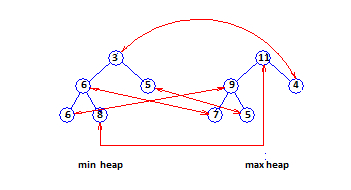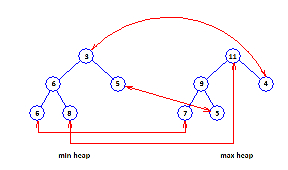# Correspondence Based Data Structures

Total and leaf correspondence are more sophisticated correspondence techniques. In both of these techniques, half the elements are located in the min PQ and the other half in the max PQ. When the number of elements is odd, one element is stored in a buffer. This buffered element is not the member of either PQ. In total correspondence technique, each element x in the min PQ is paired with a distinct element y of the max PQ. (x, y) is a corresponding pair of elements such that priority(x) <= priority(y).

Figure E displays a total correspondence heap for the 11 elements 3, 4, 5, 5, 6, 6, 7, 8, 9, 10, 11. The element 10 is in the buffer. Corresponding pairs are displayed by red arrows.Figure E: Total correspondence heap

In leaf correspondence technique, each leaf element of the min and max PQ is needed to be part of a corresponding pair. Non-leaf elements do not require to be in any corresponding pair. Figure F displays a leaf correspondence heap.Figure F: A leaf correspondence heap

Total and leaf correspondence structures need less space than dual structures. However, the DEPQ Algorithms for total and leaf correspondence structures are more complicated than those for dual structures. Of the three correspondence techniques, leaf correspondence is the fastest DEPQ correspondence structures.